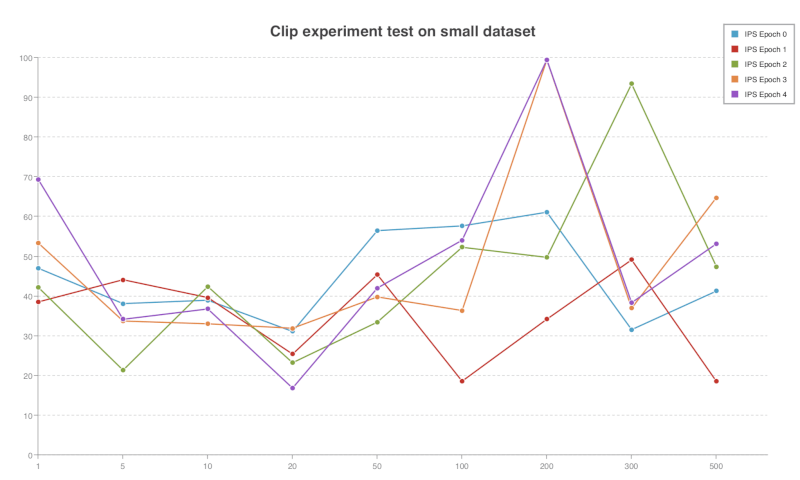Weekly ReportJinning Li Aug 02, 2018

# Weekly Report 

Jinning, 08/02/2018

## Propensity weighted BCE loss

Run experiment with both propensity weighted or not. All the two result are based on same hyperparameters:

• Non-Propensity (weight: 1) Epoch 10: IPS: 54.5463082902, IPS_std: 2.943

• propensity (weight: 1/propensity) Epoch 10: IPS: 55.1079999611, IPS_std: 6.328

#### Intuition:

Introducing propensity can improve the performance of LR model slightly. Overfitting will probably happen at epoch 10. It’s possible that propensity can reduce the bias and relieve overfitting.

## Clip Experiment

Clip propensity weighted BCE loss. This means the weight applied to BCE loss is not simply $\frac{1}{p}$, but $\min\{\frac{1}{p}, c\}$, where $c$ is a constant. In my experiment, $c$ is selected as 1, 5, 10, 20, 50, 100, 200, 300, 500.

When $c=1$, this is equivalent to unweighted BCE. When $c=500$, since $\frac{1}{p}$ is less than $500$, this is equivalent to $\frac{1}{p}\times loss$.

Result:Note that this experiment is trained on full training set and test on small test set.
Not so reliable.

The highest IPS appears around $c=200$. So I guess the best $c$ is the average or median of all the propensity values.